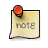# Development Highlight: New Settings System

As a preview of a feature available in the nighty builds, a large change was made to how KiCad stores and loads settings that not only fixes a few headaches with the old system but lays the groundwork for more customization in KiCad such as color themes. This was thanks to the work of Jon Evans in MR#68

## New Settings Save Location and Wizard

Settings are now stored on a versioned basis on all platforms.

For example, settings on Windows used to be stored only in `%APPDATA%\kicad` and all versions of KiCad thus far wanted to write to the exact same versions. This resulted in headaches when upgrading KiCad versions as older config files sometimes had bad settings for the newer version.

With this change, settings are now stored in `%APPDATA%\kicad\<version>\`

Additonally, there is now a upgrade wizard that will open if you are opening a version of KiCad for the first time.

## Settings File Format Change

Almost all settings are now stored as JSON formatted files instead of the wxWidgets based ini file format. This aids greatly in making complex setting objects more developer friendly to implement than before and opens the path for third party tools to read the settings more easily if so desired.

Old format example

``````KicadFramePos_x=0
KicadFramePos_y=0
KicadFrameSize_x=759
KicadFrameSize_y=400
KicadFrameMaximized=0
KicadFramePerspective=layout2|name=MainToolbar;caption=;state=139644;dir=1;layer=6;row=0;pos=0;prop=100000;bestw=273;besth=36;minw=-1;minh=-1;maxw=-1;maxh=-1;floatx=-1;floaty=-1;floatw=-1;floath=-1|name=ProjectTree;caption=;state=508;dir=4;layer=3;row=0;pos=0;prop=100000;bestw=60;besth=-1;minw=150;minh=-1;maxw=-1;maxh=-1;floatx=-1;floaty=-1;floatw=-1;floath=-1|name=Launcher;caption=;state=139644;dir=1;layer=1;row=0;pos=0;prop=100000;bestw=525;besth=70;minw=525;minh=70;maxw=-1;maxh=-1;floatx=-1;floaty=-1;floatw=-1;floath=-1|name=MsgPanel;caption=;state=131452;dir=5;layer=0;row=0;pos=0;prop=100000;bestw=101;besth=30;minw=-1;minh=-1;maxw=-1;maxh=-1;floatx=-1;floaty=-1;floatw=-1;floath=-1|dock_size(1,6,0)=36|dock_size(4,3,0)=150|dock_size(1,1,0)=70|dock_size(5,0,0)=101|
KicadFrameMostRecentlyUsedPath=C:\\Users\\kicaduser\\Documents
LeftWinWidth=150
file1=
file2=
file3=
file4=
file5=
file6=
file7=
file8=
file9=``````

New format example

``````{
"appearance": {
"color_theme": "user",
"left_frame_width": 200
},
"find_replace": {
"find_history": [],
"find_string": "",
"flags": 1,
"replace_history": [],
"replace_string": ""
},
"graphics": {
"canvas_type": 2
},
"lib_tree": {
"column_width": 360
},
"meta": {
"filename": "kicad",
"version": 0
},
"printing": {
"layers": [],
"monochrome": true,
"scale": 1.0,
"title_block": false
},
"system": {
"file_history": [],
"first_run_shown": false,
"max_undo_items": 0,
"units": 1
},
"window": {
"cursor": {
"always_show_cursor": true,
"fullscreen_cursor": false
},
"grid": {
"axes_enabled": false,
"last_size": 0,
"line_width": 1.0,
"min_spacing": 10.0,
"show": true,
"style": 0
},
"maximized": true,
"mru_path": "",
"perspective": "layout2|name=MainToolbar;caption=;state=139644;dir=1;layer=6;row=0;pos=0;prop=100000;bestw=291;besth=39;minw=-1;minh=-1;maxw=-1;maxh=-1;floatx=-1;floaty=-1;floatw=-1;floath=-1|name=ProjectTree;caption=;state=508;dir=4;layer=3;row=0;pos=0;prop=100000;bestw=200;besth=-1;minw=200;minh=-1;maxw=-1;maxh=-1;floatx=-1;floaty=-1;floatw=-1;floath=-1|name=Launcher;caption=;state=139644;dir=1;layer=1;row=0;pos=0;prop=100000;bestw=496;besth=61;minw=-1;minh=-1;maxw=-1;maxh=-1;floatx=-1;floaty=-1;floatw=-1;floath=-1|name=MsgPanel;caption=;state=131452;dir=5;layer=0;row=0;pos=0;prop=100000;bestw=101;besth=30;minw=-1;minh=-1;maxw=-1;maxh=-1;floatx=-1;floaty=-1;floatw=-1;floath=-1|dock_size(1,6,0)=39|dock_size(4,3,0)=200|dock_size(1,1,0)=61|dock_size(5,0,0)=101|",
"pos_x": -8,
"pos_y": -8,
"size_x": 1936,
"size_y": 1066
}
}``````Both this feature and nightly builds are in development, please only use them for testing and experimentation